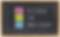# Annualized Return Formula: Calculate Your ROI

## How to accurately calculate return on your investmentsOne of the best indicators of how your investments are doing is the annual return percentage (APY = Annual Percentage Yield). The APY takes into account not only your return, but how long it took you to get that return. Learn how to make sense of your investment statements and find out if you’re really getting the “market beating” returns your financial advisor insists you are. If, for example, we turn a \$10,000 investment into \$20,000 in five years, we’re much better off, both because of inflation and present value, then we are if it takes us ten years to garner the same return.

We're guessing the whole reason you found this page is so you can find the formula necessary for computing annualized return. Well, we don't want to keep you waiting.

## Annualized Return Formula

APY = ((principal + gain) / principal) ^ (365/days) - 1

So, for example, suppose our initial investment (ie. principal) is \$10,000, and after 2.5 years we are sitting on \$14,000. What is our annual return? Let's plug our numbers into our formula using the following values:

• principal = \$10,000
• gain = \$4,000
• days = (365 * 2.5) = 913 (rounding our half-day up)

APY = (\$14,000 / \$10,000) ^ (365 / 913) - 1 = 14%

### Rounding off the years

If you don't need to calculate your return to the day (which you often would unless you invested on January 1st), you can use years to calculate your APY:

APY = (principal + gain/principal) ^ (1/years) - 1

For example:

APY = (\$14,000 / \$10,000) ^ (1/2.5) - 1 = 14%

Our annualized return in this case is 14%. Not bad, right? If we take the numbers by themselves we ended up with more than we started with, which is a good thing. And we earned more than the rate of inflation over those 913 days, so our money is definitely worth more than it was before. The real question is, when did we invest this money? If we invested in a bull market, the return is not all that surprising. If, on the other hand, we invested in a bear market, we now look like a Wall Street maverick. By comparing our return to the market, we are able to gauge how effective our investment selections were relative to how everyone else is doing. Our market index comparison of choice is the S&P 500 - a collection of 500 large-cap, U.S.-based companies put together by Standard & Poors, a division of McGraw-Hill.

## Projecting your returns into the future

Just how much will your money compound and grow into the future using this calculation? Let's say you want to know how much your \$14,000 will be worth in 10 or 25 years if it continues to grow at a 14% clip? Since we have the value for APY, we simply re-arrange the equation to find our new missing ending value, or projected return (PR):

PR = (principal + gain) (1 + APY)Y

where Y = # years

Let's take an example. Let's find out what our \$10,000 investment will be worth after 25 years with an annual return of 14%:

PR = (\$10,000 + \$4,000)(1 + 14%)25 = \$370,466.82.

Whew, not bad, eh? And that's assuming we don't add any more funds to this investment. The best way to invest is to set aside some money each month to invest. That will really help your portfolio compound and grow.

### Compounding more than once per year

For those that want to get even more refined, here's a calculation where you can define the number of times you compound per year (the above formula assumes you compound once per year):

PR = (principal + gain) (1 + APY/n)Y/n

where n = # times you compound per year